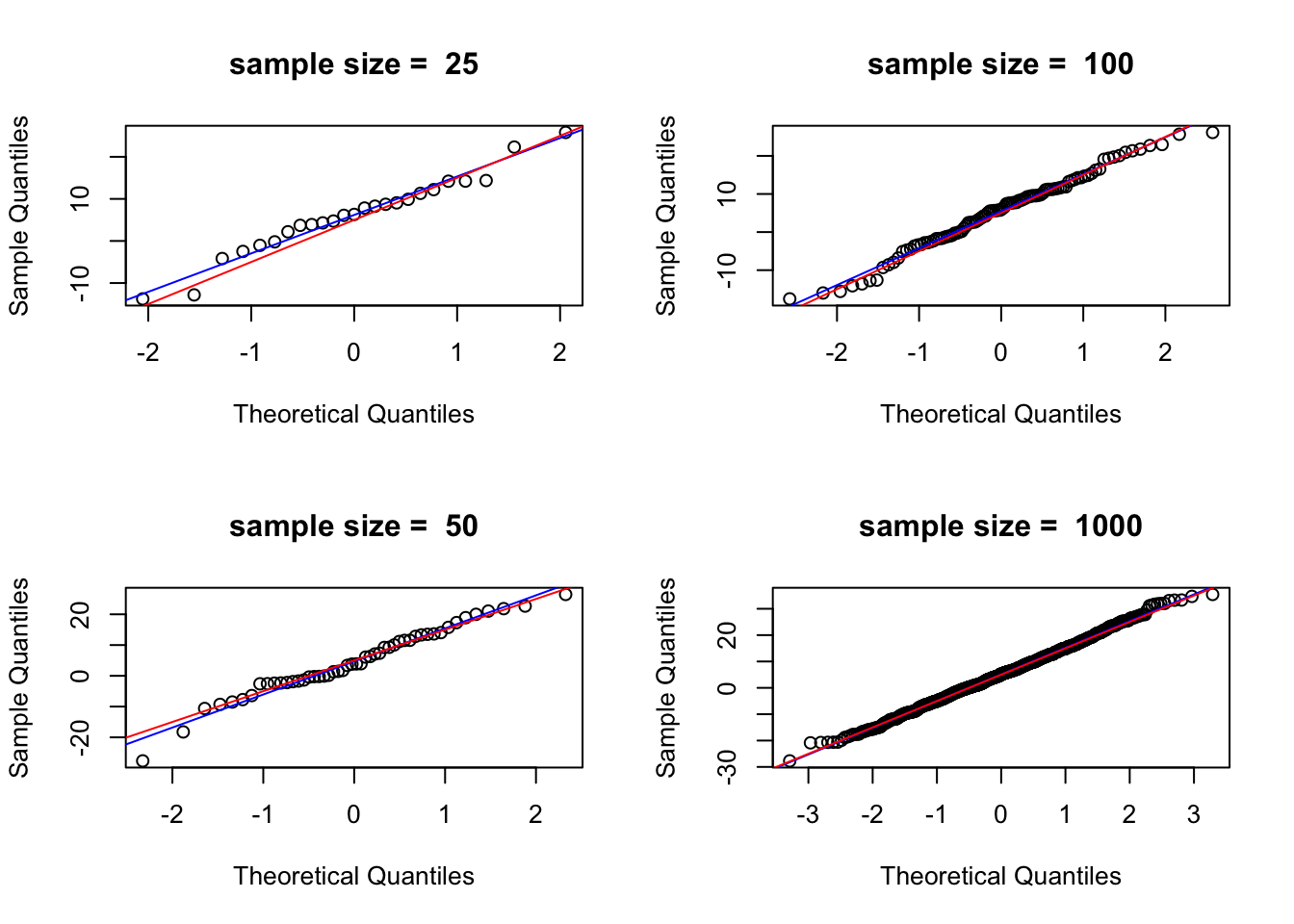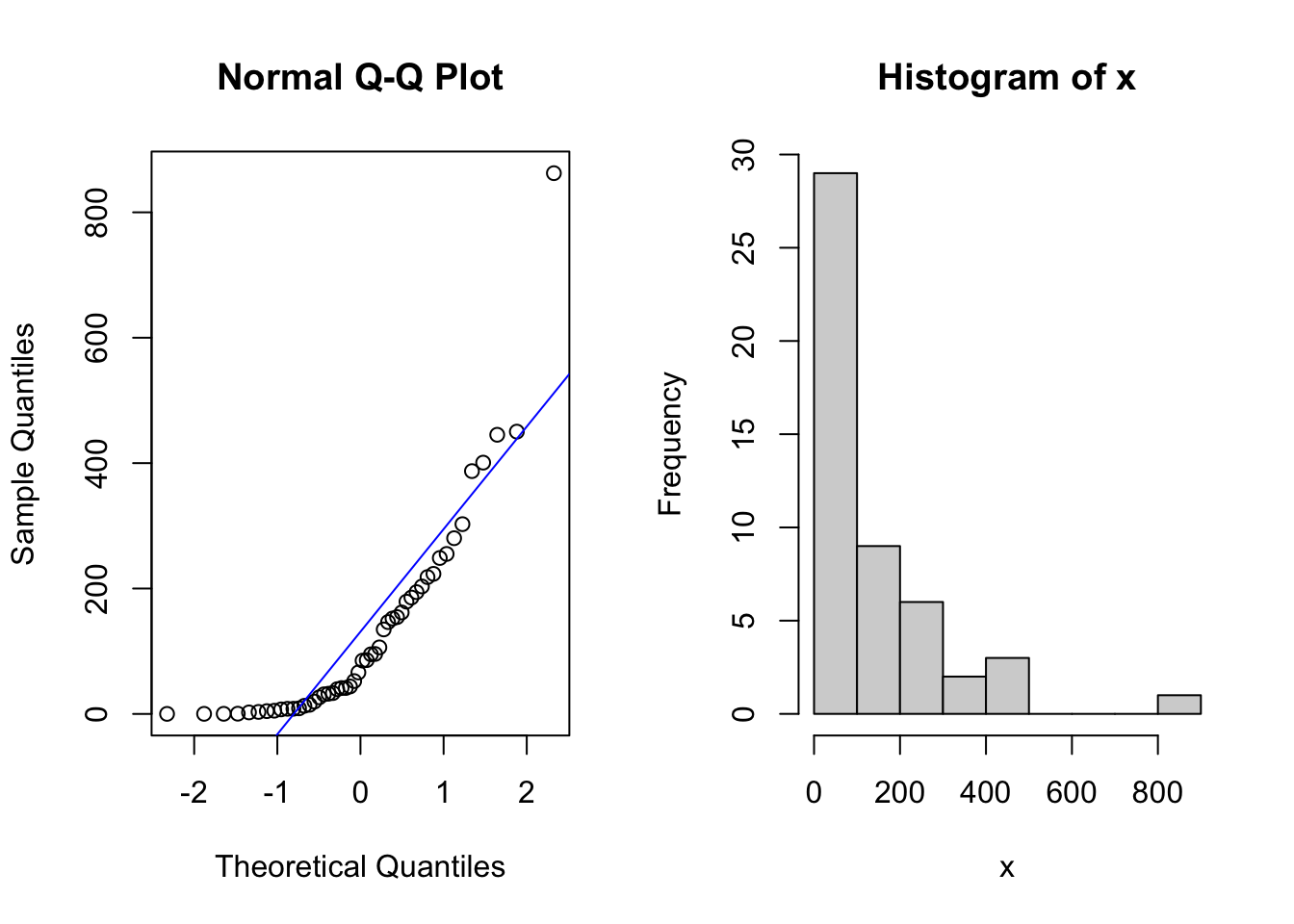# Chapter 1 Recall on Q-Q plot

par(mfcol = c(2,2))
for (n in c(25,50,100,1000)){
x = rnorm(n, mean = 5, sd = 10)
qqnorm(x,main=paste("sample size = ",n))
abline(mean(x), sqrt(var(x)), col = 'blue')
abline(5, 10, col = 'red')
}par(mfcol = c(1,2))
x = rnorm(50, mean = 5, sd = 10)
x = x^2
qqnorm(x)
abline(a = mean(x), b = sqrt(var(x)), col = 'blue') # sample
hist(x)